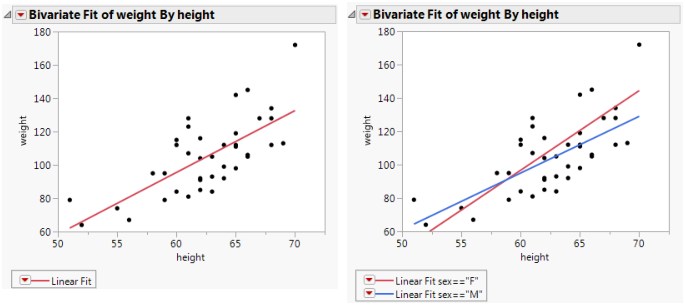Basic Analysis > Bivariate Analysis > Additional Examples of the Bivariate Platform > Example of Group By Using Regression Lines
Publication date: 04/12/2021

## Example of Group By Using Regression Lines

This example shows you how to use a grouping variable to overlay regression lines to compare slopes of the different groups.

1. Select Help > Sample Data Library and open Big Class.jmp.

2. Select Analyze > Fit Y by X.

3. Select weight and click Y, Response.

4. Select height and click X, Factor.

5. Click OK.

To create the example on the left in Figure 5.24:

6. Click the red triangle next to Bivariate Fit of weight By height and select Fit Line.

To create the example on the right in Figure 5.24:

7. From the Linear Fit menu, select Remove Fit.

8. Click the red triangle next to Bivariate Fit of weight By height and select Group By.

9. From the list, select sex.

10. Click OK.

11. Click the red triangle next to Bivariate Fit of weight By height and select Fit Line.

Figure 5.24 Example of Regression Analysis for Whole Sample and Grouped SampleThe scatterplot to the left in Figure 5.24 has a single regression line that relates weight to height. The scatterplot to the right shows separate regression lines for males and females.

Want more information? Have questions? Get answers in the JMP User Community (community.jmp.com).